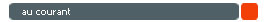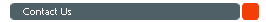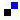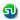Home About Us Practice Test GMAT eBooks Math Books GMAT Classes Forums B SchoolsGMAT Frequently Asked Questions GMAT CAT Test Details / Signup GMAT Syllabus, Testing Subject areas GMAT Math Syllabus, Sample QuestionseBook on Number Theory and Inequalities eBook on Permutation & Combination eBook on Geometry Doubts, discussions in GMAT Math Share your GMAT Test experience 4GMAT's Achievers' List TestimonialsFree GMAT Questions Answers Free GMAT Math Test GMAT Blogs GMAT Quant Blogs GMAT FAQsTop US B Schools R1/R2 deadlines Featured B School Rearranging numbers - Permutation Counting number of 1s and 0sChennai : 95000 48484 Mumbai : 80970 48484 Bangalore : 91419 48484 eMail : info@4gmat.comYou are here: 4GMAT Home » GMAT FAQ » GMAT Quant Section » GMAT Data Sufficiency (DS)

# Quantitative Reasoning - GMAT Data Sufficiency (DS)

Data-Sufficiency questions in the GMAT test measure a candidate's proficiency in the same principles and areas of Math that are measured by the GMAT problem solving questions.

However, the pattern and specific nature of the data sufficiency questions are designed to measure your ability to:
• analyze a quantitative problem and recognize which information is relevant and
• determine what information is sufficient to solve a given problem
This is how a Sample Data Sufficiency Question in GMAT looks like.

#### Directions

This data sufficiency problem consists of a question and two statements, labeled (1) and (2), in which certain data are given. You have to decide whether the data given in the statements are sufficient for answering the question. Using the data given in the statements, plus your knowledge of mathematics and everyday facts (such as the number of days in a leap year or the meaning of the word counterclockwise), you must indicate whether -
1. Statement (1) ALONE is sufficient, but statement (2) alone is not sufficient to answer the question asked.
2. Statement (2) ALONE is sufficient, but statement (1) alone is not sufficient to answer the question asked.
3. BOTH statements (1) and (2) TOGETHER are sufficient to answer the question asked, but NEITHER statement ALONE is sufficient to answer the question asked.
5. Statements (1) and (2) TOGETHER are NOT sufficient to answer the question asked, and additional data specific to the problem are needed.
Numbers

All numbers used are real numbers.

Figures

A figure accompanying a data sufficiency question will conform to the information given in the question but will not necessarily conform to the additional information given in statements (1) and (2).

Lines shown as straight can be assumed to be straight and lines that appear jagged can also be assumed to be straight.

You may assume that the positions of points, angles, regions, etc. exist in the order shown and that angle measures are greater than zero.

All figures lie in a plane unless otherwise indicated.

Note: In data sufficiency problems that ask for the value of a quantity, the data given in the statement are sufficient only when it is possible to determine exactly one numerical value for the quantity.

#### Question

If x and y are non-zero numbers, is x = y?
1. (x - 25)2 = (y - 25)2
2. x/y = y/x

Correct answer is choice (C). BOTH statements (1) and (2) TOGETHER are sufficient to answer the question asked, but NEITHER statement ALONE is sufficient to answer the question asked.

The question asks us to evaluate if x = y.

Statement I
From statement 1, we know that (x - 25)2 = (y - 25)2.
This could have two possible situations. One that x = y. The second one is possible when let us say x takes the value of 50 and y takes the value of '0'. i.e., x is not equal to y. As we are not able to conclusively decide if x is equal to y or not, statement I alone is not sufficient.

We can therefore, rule out answer choices A and D. The answer has to be one of B, C or E.

Statement II
From statement II, we can conclude that x2 = y2.
This translates to x2 - y2 = 0 or (x + y)(x - y) = 0
or at least one of (x + y) or (x - y) = 0
or x = -y or x = y.
From statement II also we are not able to conclusively decide if x is equal to y. Therefore, statement II alone is not sufficient.
We can eliminate choice B also. So, the answer has to be either C or E.

If we combine the information in both the statements, this is how the expansion of the first statement will look
x2 - 50x + 625 = y2 - 50x + 625
or x2 - 50x = y2 - 50y
From statement II, we know that x2 = y2.
Therefore, x2 - 50x = y2 - 50y can be simplified as -50x = -50y
or x = y.

As we can conclude that x = y using information in both the statements, the correct answer is choice (C).Add to del.icio.usStumble Itdigg this